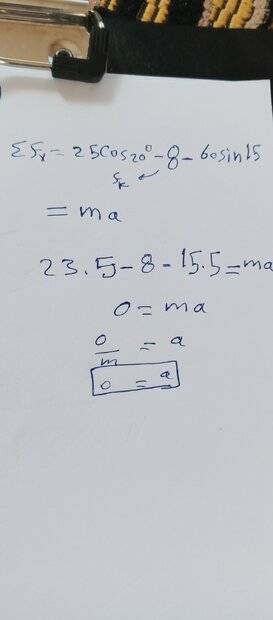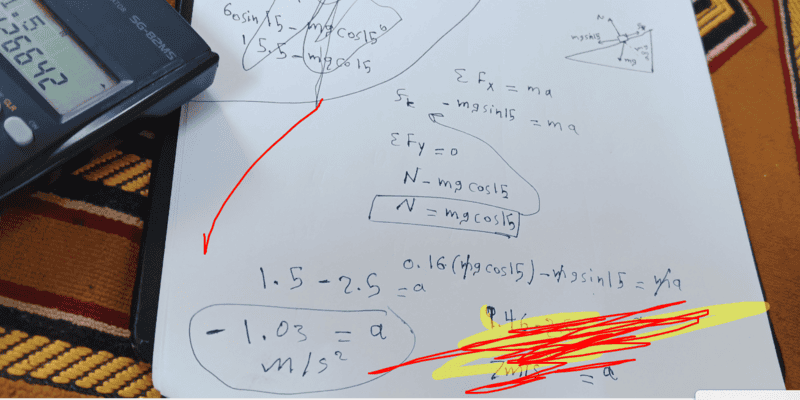# Help me with this boy who is dragging a skate up a hill

Oh lord oh no! yes you are right 🤦Thanks guys now I can see how the mass cancel and I got the accelerationf

Homework Statement
A boy drags his 60N sled at constant speed up a 15 degree hill. He does so by pulling with a 25N force on a rope attached to the sled. If the rope is inclined at 35 degrees to the horizontal

What is the coefficient of kinetic friction between the sled and snow?

At the top of the hill he jumps on the sled and slides down the hill. What is the magnitude of his acceleration down the slope.
----------------------------
Relevant Equations
How to find a?
I've found M-k it is 0.16
how to find the acceleration? I can't solve it without the quetion telling me the mass of the kid!

Homework Statement: A boy drags his 60N sled at constant speed up a 15 degree hill. He does so by pulling with a 25N force on a rope attached to the sled. If the rope is inclined at 35 degrees to the horizontal

What is the coefficient of kinetic friction between the sled and snow?

At the top of the hill he jumps on the sled and slides down the hill. What is the magnitude of his acceleration down the slope.
----------------------------
Relevant Equations: How to find a?

I can't solve it without the quetion telling me the mass of the kid!
The masses are irrelevant for the second part. Just put an unknown for it, m, and watch it disappear.

The masses are irrelevant for the second part. Just put an unknown for it, m, and watch it disappear.
That's what I've been told and I still don't get how!
Can you please show me how so?

That's what I've been told and I still don't get how!
Can you please show me how so?
As I suggested, let the boy's mass be m and write out the equations. Use M for the sled's mass, rather than plug in numbers.
Post what you get.

•erobz and MatinSAR
Homework Statement: A boy drags his 60N sled at constant speed up a 15 degree hill. He does so by pulling with a 25N force on a rope attached to the sled. If the rope is inclined at 35 degrees to the horizontal

What is the coefficient of kinetic friction between the sled and snow?

At the top of the hill he jumps on the sled and slides down the hill. What is the magnitude of his acceleration down the slope.
----------------------------
Relevant Equations: How to find a?

I've found M-k it is 0.16
how to find the acceleration? I can't solve it without the quetion telling me the mass of the kid!
I agree with ##µ_k≈0.16## , Are you sure that the question asks for the acceleration down the slope? Or it asks for velocity?
To find the acceleration, not only the mass of the boy but also the mass of the sled is not needed. Just use Newton's second law for an object sliding down a ramp.

I agree with ##µ_k≈0.16## , Are you sure that the question asks for the acceleration down the slope? Or it asks for velocity?
To find the acceleration, not only the mass of the boy but also the mass of the sled is not needed. Just use Newton's second law for an object sliding down a ramp.
As I suggested, let the boy's mass be m and write out the equations. Use M for the sled's mass, rather than plug in numbers.
Post what you get.
I get this a =0and in the textbook the asnwer is 1 and idk how

I get this a =0
There is no tension force when the boy slides down! First try to draw a free body diagram.
Consider the x-axis along the ramp and the y-axis perpendicular to it.

•Oh lord oh no! yes you are right•